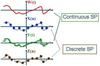Stochastic Process

• 随机过程 随机过程被认为是概率论的“动力学”部分。意思是说，它的研究对象是随时间演变的随机现象。对于这种现象，一般来说，人们已不能用随机变量或多位随机变量来合理表达，而需要用一族随机变量来描述。 设...
随机过程

随机过程被认为是概率论的“动力学”部分。意思是说，它的研究对象是随时间演变的随机现象。对于这种现象，一般来说，人们已不能用随机变量或多位随机变量来合理表达，而需要用一族随机变量来描述。

设T是一个无限实数集。我们把依赖于参数t∈T的一族（无限多个）随机变量称为随机过程，记为{X(t),t∈T}，这里对每一个t∈T，x(t)是一随机变量。T叫做参数集。我们常把t看作为时间，称X(t)为时刻t时过程的状态，而X(t1)=x(实数)说成是t=t1时过程处于状态x，对于一切t∈T，X(t)所有可能取的一切值的全体称为随机过程的状态空间。

对随机过程{X(t),t∈T}进行一次试验（即在T上进行一次全程观测），其结果是t的函数，记为x(t)，t∈T，称他为随机过程的一个样本函数或样本曲线。

例：设某城市的120急救电话台迟早会接到用户的呼叫，以X(t)表示时间间隔(0,t]内接到的呼叫次数，它是一个随机变量，且对于不同的t≥0，X(t)是不同的随机变量，于是，{X(t),t≥0}是一随机过程，且它的状态空间是[0,1,2,……}

随机过程的统计描述

随机过程的分布函数
给定随机过程{X(t),t∈T}。对于每一个固定的t∈T,随机变量X（t）的分布函数一般与t有关，记为
Fx(x,t)=P{X(t)≤x},x∈R,
称它为随机过程{X(t),t∈T}的一维分布函数，而{Fx(x,t),t∈T}称为一维分布函数族。

随机过程的数字特征

给定随机过程{X(t),t∈T}。固定的t∈T,X(t)是一随机变量，它的均值一般与t有关，记为
μx(t)=E[X(t)]
我们称为μx(t)为该随机过程的均值函数。
其次，我们把随机变量X(t)的二阶原点矩和二阶中心矩分别记作
Ψ²(t)=E[X²(t)]和σ²(t)=Dx(t)=var{X(t)}=E{[X(t)-μx(t)]}²并称他们为随机过程的均方值函数和方差函数。方差函数的算术平方根成为随机过程的标准差函数。她表示随机过程在时刻t对于均值的平均偏离程度。
泊松过程
一种累计随机事件发生次数的最基本的独立增量过程。例如随着时间增长累计某电话交换台收到的呼唤次数，就构成一个泊松过程。
泊松过程是随机过程的一种，是以事件的发生时间来定义的。我们说一个随机过程N(t) 是一个时间齐次的一维泊松过程，如果它满足以下条件：
在两个互斥（不重叠）的区间内所发生的事件的数目是互相独立的随机变量。
在区间 [t,t+τ]内发生的事件的数目的概率分布为一个泊松分布
其中λ是一个正数，是固定的参数，通常称为抵达率（arrival rate）或强度。
~~
马尔科夫链
~~
过程在时刻t0所处的状态为已知的条件下，过程在时刻t＞t0所处状态的条件分与过程在时刻t0之前所处的状态无关。通俗地说，就是在已经知道过程“现在”的条件下，其“将来”不依赖于“过去”。
现用分布函数来表述马尔科夫性。设随机过程{X(t),t∈T}的状态空间为I.如果对时间t的任意n个数值t1＜t2＜…tn,n≥3，ti∈T，在条件X(ti)=xi,xi∈I，i=1,2…,n-1下，X(tn)的条件分布函数恰等于在条件X(tn-1)=xn-1下X(tn)的条件分布函数，即

P{X(tn)≤xn|X(t1)=x1,X(t2)=x2,…,X(tn-1)=xn-1}=P{X(tn)≤xn|X(tn-1)=xn-1}xn∈R，则称该随机过程具有马尔科夫性或无后效性，并称此过程为马尔科夫过程。
高斯过程
高斯过程（Gaussian Process, GP）是概率论和数理统计中随机过程（stochastic process）的一种，是一系列服从正态分布的随机变量（random variable）在一指数集（index set）内的组合   。
高斯过程中任意随机变量的线性组合都服从正态分布，每个有限维分布都是联合正态分布，且其本身在连续指数集上的概率密度函数即是所有随机变量的高斯测度，因此被视为联合正态分布的无限维广义延伸   。高斯过程由其数学期望和协方差函数完全决定，并继承了正态分布的诸多性质
高斯过程指的是一组随机变量的集合，这个集合里面的任意有限个随机变量都服从联合正态分布     。具体地，对概率空间 （Ω，F,P）内指数集为 T的随机过程 X={Xt}t∈T，当X的子集（Xt1,…,Xtn）对任意{t1…,tn}∈T，n≥1都是高斯随机向量时，{Xt}被称为高斯过程。


展开全文• 一、随机过程的基本概念 1.随机过程---一类随时间作随机变化的过程。 2.随机过程的描述 a.随机过程是对应不同随机试验结果的时间过程的集合。 每一次的随机试验都是一个确定的时间函数 , 它是随机过程的一次...
                                      一、随机过程的基本概念

1.随机过程---一类随时间作随机变化的过程。

如何理解？

先理解随机变量：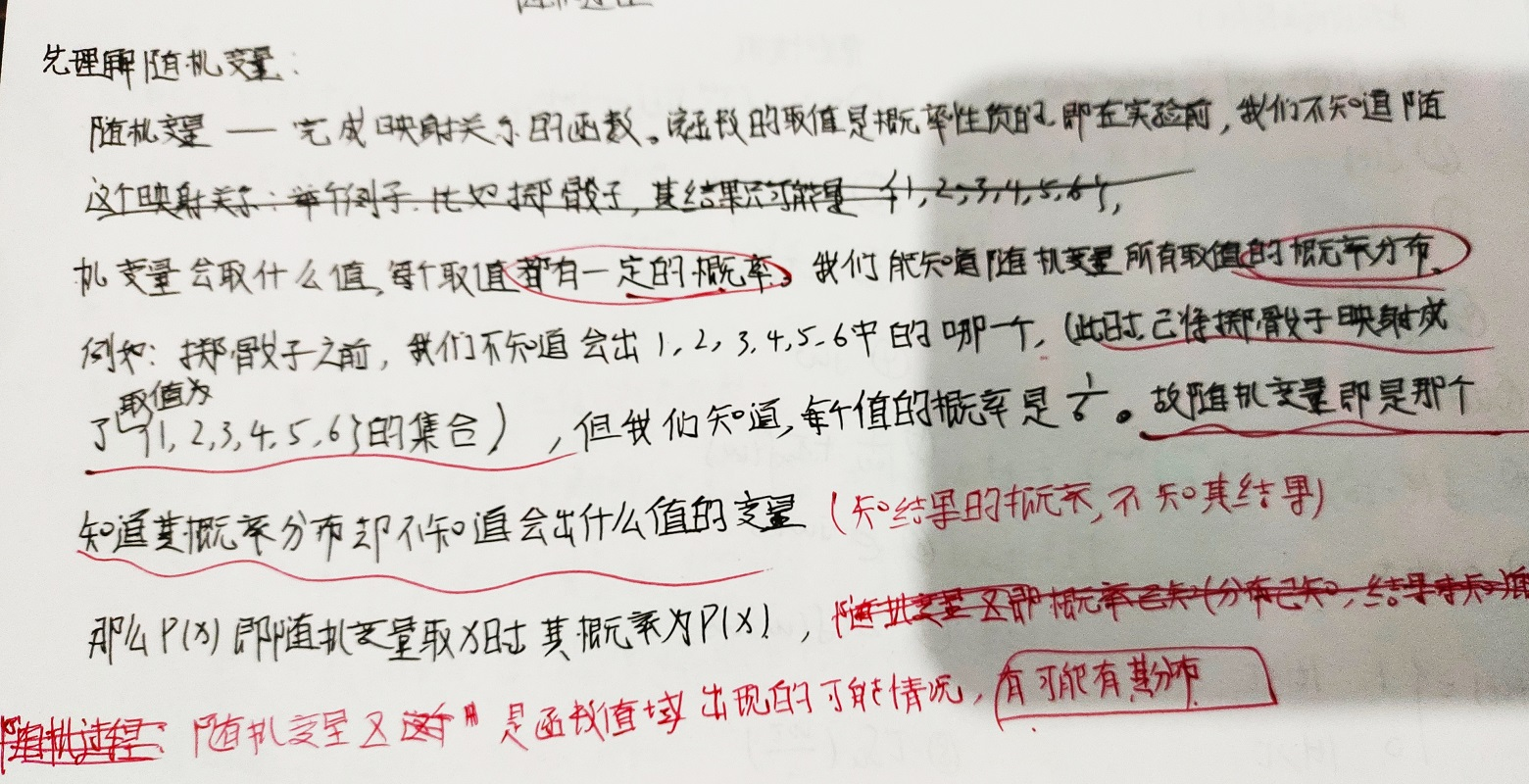再来理解随机过程：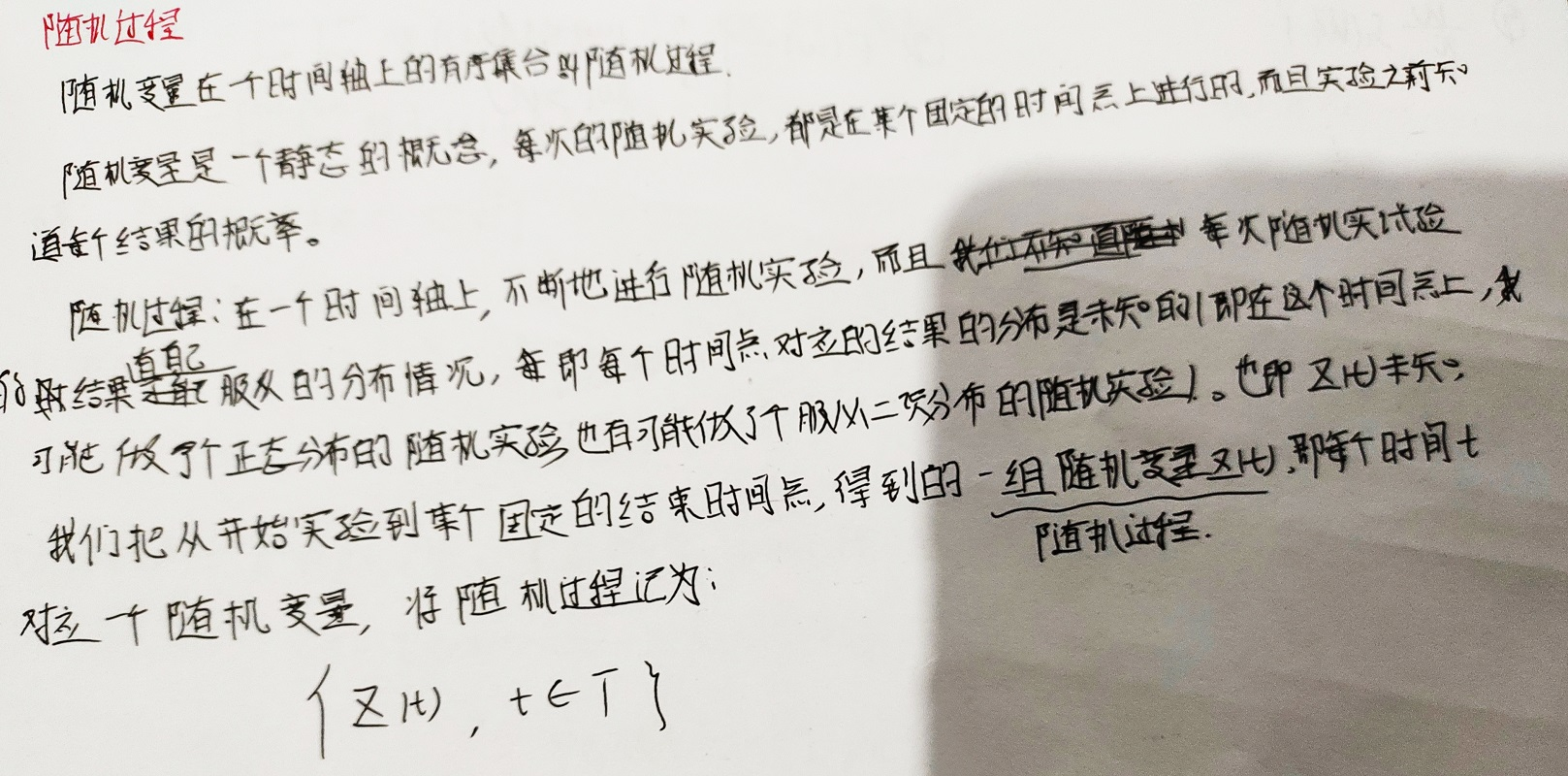2.随机过程的描述

a.随机过程是对应不同随机试验结果的时间过程的集合。

每一次的随机试验都是一个确定的时间函数  , 它是随机过程的一次实现，全体样本函数的总体就是一个随机过程。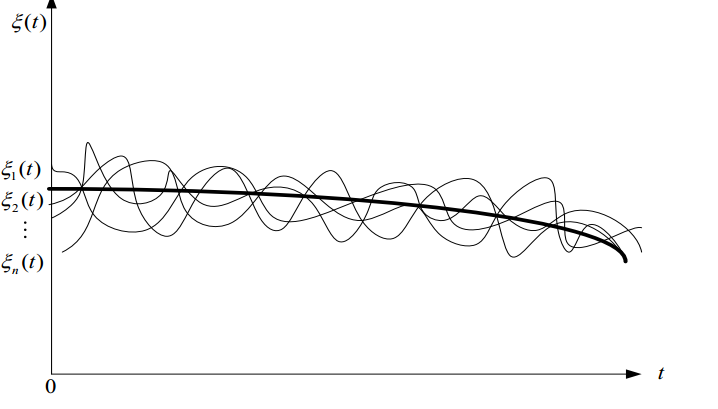b.随机过程是随机变量概念的延伸。

在一个固定时刻t1上，不同样本的取值是一个随机变量，记为。

因此，随机过程也可以看作是在时间进程中处于不同时刻的随机变量的集合。

3.随机过程的数字特征

均值：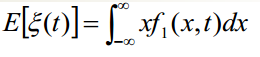是的一维概率密度函数，这个均值可以理解为在某一给定时刻t随机过程的所有样本函数的

平均值。（是在t时刻上所有样本取值的集合）的均值是时间的确定函数，记作a(t),它表示随机过程n个样本函数曲线

的摆动中心：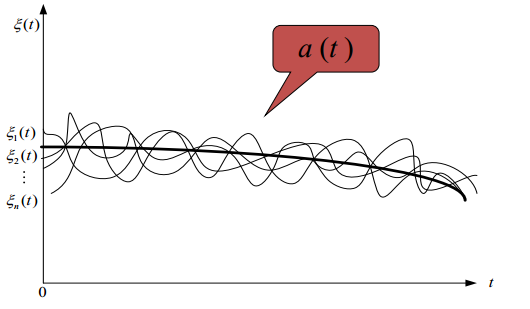方差：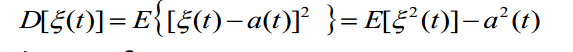记为：,它表示随机过程在时刻t 对于均值a ( t )的偏离程度。

自相关函数：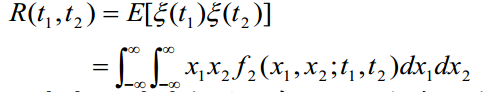和分别是在t1和t2时刻观测得到的随机变量。可以看出， R(t1, t2)是两个变量t1和t2的确定函数。互相关函数：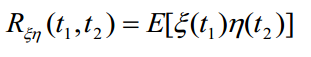式中和分别表示两个随机过程。

二、平稳随机过程

1.平稳随机过程的定义

若一个随机过程的任意有限维分布函数与时间起点无关，则称该随机过程是严平稳随机过程。

平稳随机过程的数字特征：a.均值与t无关，为常数a。

b.自相关函数只与时间间隔有关  (这相当于充分条件，判断是否是平稳，就看这俩条件)

【notice】：

同时满足a和b的过程定义为宽平稳随机过程（上述两个条件是宽平稳的充分条件）。显然，严平稳随机过程必定是宽平稳的，反之不一定成立。

在通信系统中所遇到的信号及噪声，大多数可视为平稳的随机过程。

2.各态历经性（平稳的充分条件）

含义：随机过程中的任一次实现都经历了随机过程的所有可能状态。

用处：在求解各种统计平均（均值或自相关函数等）时，只要用一次实现的时间平均”值代替过程的“统计平均”值即可，从而
使测量和计算的问题大为简化。

注意：具有各态历经的随机过程一定是平稳过程，反之不一定成立。在通信系统中所遇到的随机信号和噪声，一般均
能满足各态历经条件。

3平稳随机过程自相关函数的性质（自相关函数只与时间间隔有关）：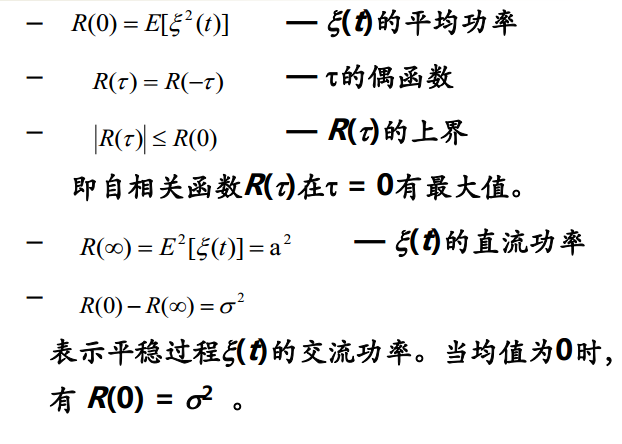4.平稳随机过程的功率谱密度

平稳随机过程的自相关函数与其功率谱密度是一对傅里叶变换。即：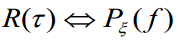在此基础上，可以得出：

a.对功率谱密度进行积分，可得平稳过程的总功率：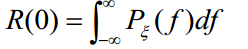b.各态历经过程的任一样本函数的功率谱密度等于过程的功率谱密度。

5.平稳随机过程通过线性系统

1）输出过程的均值

输入过程是平稳的，设其均值为a，通过线性系统，输出过程的均值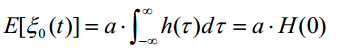其中：H(0)是线性系统在 f = 0处的频率响应，因此输出过程的均值是一个常数。

2）输出过程的自相关函数

值为：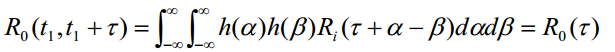只是时间间隔 的函数。由此可知，若线性系统的输入是平稳的，则输出也是平稳的。

3）输出过程的功率谱密度

输出过程的功率谱密度是输入过程的功率谱密度乘以系统频率响应模值的平方。（先求模在相乘！！！）


展开全文• 实际上联合平稳随机过程和单一的随机过程是十分相似的，联合平稳随机过程用来表征两个随机过程之间的关系。 文章目录联合平稳随机过程1.概率分布与矩函数2.矩函数3.联合平稳的矩函数 联合平稳随机过程 1.概率分布与...
实际上联合平稳随机过程和单一的随机过程是十分相似的，联合平稳随机过程用来表征两个随机过程之间的关系。
文章目录联合平稳随机过程1.概率分布与矩函数2.矩函数3.联合平稳的矩函数
联合平稳随机过程
1.概率分布与矩函数

假定有两个随机过程 $X(t)$ 和 $Y(t)$ 概率密度分别为 $p_n(x_1,x_2,\dotsb,x_n;t_1,t_2,\dotsb,t_n)$$p_m(y_1,y_2,\dotsb,y_m;t'_1,t'_2,\dotsb,t'_m)$
联合概率分布函数
$\begin{matrix} F_{m+n}(x_1,x_2,\dotsb ,x_n;y_1,y_2,\dotsb,y_m;t_1,t_2,\dotsb,t_n;t'_1,t'_2,\dotsb,t'_m)\\ ~~\\ = P\{ X(t_1) \le x_1,X(t_2) \le x_2, \dotsb X(t_n) \le x_n ;Y(t'_1) \le y_1,Y(t'_2) \le y_2, \dotsb Y(t'_n) \le y_n \} \end{matrix}$
联合概率密度函数
$\begin{matrix} p_{m+n}(x_1,x_2,\dotsb ,x_n;y_1,y_2,\dotsb,y_m;t_1,t_2,\dotsb,t_n;t'_1,t'_2,\dotsb,t'_m)\\ ~~\\ = \dfrac{\partial^n F_n(x_1,x_2,\dotsb ,x_n;y_1,y_2,\dotsb,y_m;t_1,t_2,\dotsb,t_n;t'_1,t'_2,\dotsb,t'_m)}{\partial x_1\partial x_2 \dotsb \partial x_n\partial y_1\partial y_2 \dotsb \partial y_m} \end{matrix}$
过程的统计独立：
$\begin{matrix} p_{m+n}(x_1,x_2,\dotsb ,x_n;y_1,y_2,\dotsb,y_m;t_1,t_2,\dotsb,t_n;t'_1,t'_2,\dotsb,t'_m) \\ ~~\\ = p_n(x_1,x_2,\dotsb ,x_n,t_1,t_2,\dotsb,t_n) p_m(y_1,y_2,\dotsb ,y_m,t'_1,t'_2,\dotsb,t'_n) \end{matrix}$

2.矩函数

互相关函数：
$R_X(t_1,t_2) = E[X(t_1)Y(t_2)]=\int _{-\infin}^{\infin}\int _{-\infin}^{\infin}xy~p_2(x,y;t_1,t_2)dxdy$
互协方差函数：
$C_{XY}(t_1,t_2) = E[X(t_1)-m_X(t_1)][Y(t_2)-m_Y(t_2)]$

3.联合平稳的矩函数

定义：$p_{m+n}$ 不随时间的改变而变化，称过程 $X(t)$ 和 $Y(t)$ 联合平稳。
互相关函数：$R_{XY}(t_1,t_2) = R_{XY}(\tau)$
互相关系数：$r_{XY}(\tau) = \frac{C_{XY}(\tau)}{\sigma_X\sigma_Y}$
互协方差函数：$C_{XY}(t_1,t_2) = C_{XY}(\tau)$
正交：对任意 $\tau$，$r_{XY}(\tau)$ 均为 $0$，就是说过程 $X(t)$ 和过程 $Y(t)$完全不相关（线性）。可以用 $E[X(t)Y(t+\tau)] = 0$ 来表示两过程正交。
⭐️ 注意区分独立和不相关，独立的定义要比不相关更加严格。
互相关函数性质：

$R_{XY}(\tau) = R_{YX}(-\tau)$
$|R_{xy}(\tau)|^2\le R_X(0)R_Y(0)$


展开全文人工智能 机器学习
• 随机过程（基本概念、平稳随机过程） 大三上学习了随机过程（实际听不懂就去看了简化版的随机信号），感觉一些概念性的东西比较多，因此这里进行一个简单总结。 文章目录(1)随机过程的基本概念1.对随机过程的理解2....
大三上学习了随机过程（实际听不懂就去看了简化版的随机信号），感觉一些概念性的东西比较多，因此这里进行一个简单总结。
文章目录(1)随机过程的基本概念1.对随机过程的理解2.随机过程的概率分布3.随机过程的矩函数4.随机过程的特征函数(2)平稳随机过程1.平稳的定义2.严格平稳过程3.广义平稳过程4.各态历经过程5.平稳过程相关函数的性质6.相关系数和相关时间
(1)随机过程的基本概念
1.对随机过程的理解

我感觉所谓的随机过程实际很类似升维之后的随机变量，但是横坐标是确知的时间 $t$。对每一个时间 $t$ 进行切片（降维）都会得到对应的一组随机变量，而针对每一个确定的时间 $t$ 进行观测都会得到一个确知的随机变量的取值，所有的 $t$ 对应的这个取值就会构成一个确定的时间函数（样本函数）。

2.随机过程的概率分布

一维分布函数：
$F_1(x_1,t_1) = P\{ X(t_1) \le x_1\}$
就像在理解中说的，在这里相当于每给定一个 $t_1$，二维的这样的随机过程就转换成一维的随机变量，所以这样的分布函数和在概率论中学到的分布函数在形式上是完全一样的。
一维概率密度：
$p_1(x_1,t_1) = \frac{\partial F_1(x_1,t_1)}{\partial x_1}$
一般省略脚注写作 $p(x,t)$ 。
$n$ 维分布函数与概率密度函数
分布函数： $F_n(x_1,x_2,\dotsb ,x_n,t_1,t_2,\dotsb,t_n) = P\{ X(t_1) \le x_1,X(t_2) \le x_2, \dotsb X(t_n) \le x_n \}$
概率密度函数：$p_n(x_1,x_2,\dotsb ,x_n,t_1,t_2,\dotsb,t_n) = \frac{\partial^n F_n(x_1,x_2,\dotsb ,x_n,t_1,t_2,\dotsb,t_n)}{\partial x_1\partial x_1 \dotsb \partial x_n}$
注意这里如果 $X(t_1),X(t_2),\dotsb,X(t_n)$ 统计独立，上面的概率密度函数就可以直接乘开 $p(x_1,x_2,\dotsb ,x_n,t_1,t_2,\dotsb,t_n) = p(x_1,t_1)p(x_2,t_2)\dotsb p(x_n,t_n)$

3.随机过程的矩函数

数学期望（统计均值，一阶原点矩）
$E[X(t)] = \int _{-\infin}^{\infin} xp(x,t)dx = m_X(t)$
反映的是在 $t$ 给定条件下的样本函数的平均取值。
方差 （二阶中心矩）
$\begin{matrix} &D[X(t)]=E\{[X(t) - m_X(t)]^2 \}\\ ~~\\ &=\displaystyle\int _{-\infin}^{\infin} (x-m_X(t))p(x,t)dx=\sigma^2_X(t) \end{matrix}$

标准差实际上就是方差的平方根，即 $\sigma_X(t)$。
反映的是在 $t$ 给定条件下的样本函数和样本函数平均取值之间的偏差。

自相关函数（相关函数，二阶混合原点矩）
$R_X(t_1,t_2) = E[X(t_1)X(t_2)]=\int _{-\infin}^{\infin}\int _{-\infin}^{\infin}x_1x_2p_2(x_1,x_2;t_1,t_2)dx_1dx_2$

反映过程 $X(t)$ 在任意两时刻的取值之间的平均关联程度。
当 $t_1=t_2=t$ 时，实际上自相关函数就转化成均方值($E[X^2(t)]$)。

协方差函数
$\begin{matrix} &C_X(t_1,t_2) = E[X(t_1)-m_X(t_1)][X(t_2)-m_X(t_2)]\\ ~~\\ &=\displaystyle\int _{-\infin}^{\infin}\int _{-\infin}^{\infin}[x_1-m_X(t_1)][x_2-m_X(t_2)]p_2(x_1,x_2;t_1,t_2)dx_1dx_2\end{matrix}$

反映过程 $X(t)$ 在任意两时刻的起伏值之间的平均关联程度。
实际上这里感觉类似于有一种对速度的描述，既要从 $v$ 这个角度对速度进行描述，还要从 $a$ 加速度这个角度对速度进行描述，加速度表示的实际上就是速度的起伏变化关系。
当 $t_1=t_2=t$ 时，实际上协方差就化简成了方差。

自相关函数和协方差函数之间的关系：
$C_X(t_1,t_2) = R_X(t_1,t_2)-m_X(t_1)m_X(t_2)$

4.随机过程的特征函数

特征函数的用途：
这个实际上有点类似于时域和频域之间的一种转化，时域上困难的问题转换到频域上就变得简单许多了。这里的特征函数可以简化概率密度的求解和随机变量数字特征的求解。还要注意一下，特征函数实际上与概率密度函数构成一组傅里叶变换。
一维特征函数的定义：
$\left\{ \begin{matrix} &\Phi_X(\lambda,t) = E[e^{j\lambda X(t)}]=\displaystyle\int _{-\infin}^{\infin} e^{j\lambda x} p(x,t)dx\\ &p(x,t) = \dfrac{1}{2\pi}\displaystyle\int _{-\infin}^{\infin} \Phi_X(\lambda,t) e^{-j\lambda x}d\lambda \end{matrix}\right.$
求原点矩（对特征函数两端求 $n$ 阶导）：
$E[X^n(t)] = \int _{-\infin}^{\infin} x^np(x,t)dx=j^{-n}[\frac{\partial^n}{\partial\lambda^n}\Phi_X(\lambda,t)]_{\lambda=0}$

(2)平稳随机过程
1.平稳的定义

实际上是采用平稳的这个定义想办法将随机过程中的时间这个参量进行了弱化，对于严平稳来说一维特性（一维概率密度、均值、方差）都和时间没有关系，二维特向仅与时间差有关（二维概率密度、相关函数、协方差函数）。对于广义平稳来说，均值与时间无关，相关函数仅与时间差有关。

2.严格平稳过程

一维特性与时间无关：
$p_1(x_1,t_1)=p_1(x_1)，E[X(t)] = m_X，D[X(t)] = \sigma_X^2$
二维特性仅与时间差相关：
$\begin{matrix} &p_2(x_1,x_2;t_1,t_2)=p_2(x_1,x_2;\tau)，R_X(t_1,t_2) = R_X(\tau)\\ ~~\\ &C_X(t_1,t_2) = R_X(\tau) - m_X^2\end{matrix}$

3.广义平稳过程

这里比严格平稳过程要宽松很多，广义平稳过程仅要求均值和相关函数的特性。
$\left\{ \begin{matrix} &E[X(t)] = m_X\\ &R_X(t_1,t_2) = R_X(\tau) \end{matrix}\right.$

4.各态历经过程

统计平均
统计平均是在样本轴上来看，这个要求的是在同一时刻进行大量样本的取值再求平均，实际上在操作起来这样是很麻烦的，因为要进行十分多次的重复试验。
$\begin{matrix} &E[X(t)] = m_X\\ &R_X(t_1,t_2) = R_X(\tau) \end{matrix}$
时间平均
时间平均是在时间轴上来看的，这个只要求在众多样本中取出一个函数然后进行积分求平均就可以了,比如对某一样本函数 $x_i(t)$
时间均值 $=\lim_{T\rightarrow\infin}\frac{1}{2T}\displaystyle\int_{-T}^{T}x_i(t)dt$
时间自相关函数
$ = \lim_{T\rightarrow\infin}\frac{1}{2T}\displaystyle\int_{-T}^{T}x_i(t)x_i(t+\tau)dt$
各态历经性（遍历性）
如果 xx 的时间平均和 xx 的统计平均在概率上是相等的，就说 xx 具有遍历性。
比如如果有 $ \xlongequal{P}E[X(t)]$就说均值具有遍历性。
遍历过程：

定义：均值和相关函数都具有遍历性。
理解：实际上遍历性说明了一个样本在足够长的时间内经历了这个随机过程全部的可能状态，因此对于每一个样本都可以作为了解这个随机过程的一个典型，所以直接对时间取平均就可以了。

遍历性的充要条件：

平稳过程 $X(t)$ 的均值 $m_X$ 具有遍历性的充要条件：
$\lim_{T\rightarrow\infin}\frac{1}{2T}\displaystyle\int_{-2T}^{2T}(1-\frac{|\tau|}{2T})C_X(\tau)d\tau = 0$
平稳过程 $X(t)$ 的相关函数 $R_X(\tau)$ 具有遍历性的充要条件：
$\lim_{T\rightarrow\infin}\frac{1}{2T}\displaystyle\int_{-2T}^{2T}(1-\frac{|\alpha|}{2T})C_{\phi}(\alpha)d\alpha = 0$
这里 $\phi(t) = X(t)X(t+\tau)$
对于零均值平稳正态随机过程 $X(t)$
$\lim_{|\tau|\rightarrow\infin}C_X(\tau) = 0$
实际上这个式子想表达的意思是，在两个随机变量相差足够长的时间之后，两个随机变量之间不存在相关性，这样才能完成遍历。

5.平稳过程相关函数的性质

相关函数是时间差的偶函数：$R_X(\tau) = R_X(-\tau)$
相关函数在 $\tau = 0$ 的时刻一定为非负值：$R_X(0) \ge 0$
相关函数在 $\tau = 0$ 的时刻为最大值（就是说自己和自己相关性最高）：$R_X(0)\ge|R_X(\tau)|$
如果平稳随机不含周期分量（在相差时间无限长的时候，两者以均值形式相关）：$R_X(\infin) = m_X^2$
对周期性随机过程其相关函数也具有周期性：$R_X(\tau) = R_X(\tau+T)$
$R_X(\tau)$ 为非负定函数，即对任意数组 $t_1,t_2,\dotsb,t_n$ 和任意函数 $f(x)$，均有 $\displaystyle\sum_{i,j=1}^n R_X(t_i-t_j)f(t_i)f(t_j)\ge0$

6.相关系数和相关时间

相关系数（实际上就是进行了一个归一化处理）
$r_X(\tau) = \dfrac{R_X(\tau)-R_X(\infin)}{R_X(0)-R_X(\infin)}=\frac{C_X(\tau)}{C_X(0)}=\dfrac{C_X(\tau)}{\sigma_X^2}$
相关时间：
可以从上面的等式看出来，当 $\tau\rightarrow\infin$ 时，$r_X(\tau)\rightarrow 0$，但是工程上没有办法实现 $\infin$ 这一概念，因此规定从 $r_X(\tau) = 1$ 下降到某个很小的数（在这个数之后直接认为不相关）花费的时间为相关时间。下面给出一个衡量相关时间长短的积分式：$\tau_0 = \int_0^{\infin}r_X(\tau)d\tau$

相关时间越长表示随机过程的起伏变化越慢。


展开全文机器学习 深度学习 矩阵
• 1.一个宽平稳过程不一定是严...例2：服从柯西分布的随机变量序列是严平稳随机过程，但不是宽平稳随机过程。 2.宽平稳过程定只涉及与一维、二维分布有关的数字特征，所以一个严平稳过程只要二阶矩存在，则必定是宽平
• 一、随机过程基本概念 随机过程是一类随时间作随机变化的过程，它不能用确切的时间函数描述，可以从两个不同的角度来说明：1.是把随机过程看成所有样本函数的集合。2.把随机过程看成是在时间进程中处于不同时刻的...
• 1 随机过程的概念 2 随机过程的统计描述 3 泊松过程及维纳过程人工智能 机器学习 数学
• 随机过程电子书 大家可以看看 计算机模型建立的好书 应该认真学习学习 看看里边的内容 我在博士阶段学习的
• 随机过程随机过程是随机变量在时间域的延生。就好比，在一个时间区间内，每一个时刻的随机变量的总体，就是一个随机过程。例如，通信系统中的噪声就是一个典型的随机过程。每一个时刻都是一个随机变量，总体起来的...
• 文章目录1.1 概率空间1.2 随机变量1.3随机变量函数的分布1.4随机变量的数字特征1.5随机过程的定义和统计描述1.6 随机过程分布律和数字特征1.7 复随机过程1.8 随机过程基本类型
• 一、高斯随机过程 高斯过程也称正态随机过程，在实践中观察到的大多数噪声都是高斯型的。 （1）定义 若随机过程ξ(t)任意n维分布都服从正态分布，则称它为高斯随机过程 （2）重要性质 1.若广义平稳，则狭义平稳 2.若...
• 随机过程课后题答案，随机过程课后题答案，随机过程课后题答案，重要事情说三遍
• ## 高斯随机过程

千次阅读 2019-07-28 23:10:23
随机过程包含平稳过程，随机过程可以用分布函数和概率密度函数描述，但是比较复杂，所以我们可以用均值和方差描述随机过程，而平稳过程包含严平稳过程，而平稳过程满足一定的条件就具有各态历经性 高斯过程：随机...通信原理
• 随机过程中文版 Ross 重要的随机过程学习资源 下载必有收获
•机器学习
• title: 应用随机过程笔记（一）：随机过程的定义 date: 2019-12-23 12:16:13 mathjax: true tags: 应用随机过程 数学 categories: 数学 随机过程的定义 一个随机过程，即是一族随机变量，即对于每一个t∈Tt \in ...数据分析 数学建模 统计学 人工智能 算法
• ## 随机过程简介

万次阅读 多人点赞 2019-02-17 15:56:19
随机过程的基本概念 随机变量回顾 在进入随机过程之前，先复习一下随机变量，这也是初学概率论时经常搞不明白的地方。 个人认为，数学最本质的特征是抽象，也就是说一切事物都可以通过某种法则映射到数上，然后再来...
• 严平稳随机过程（strictly stationary process）与宽平稳随机过程（wide-sense/weakly stationary process）之间没有直接的子集关系。 严平稳随机过程定义为：“任何n维分布函数或概率密度与时间起点无关的随机...
• 文章目录正态随机过程1.定义2.概率密度3.特征函数平稳随机过程的谱分析1.能量谱与功率谱2.功率谱密度与相关函数的关系白噪声 正态随机过程 1.定义 随机过程 X(t)X(t)X(t) 的任意 nnn 维（任取 nnn 个时间点）联合...机器学习 信号处理 算法 数字信号处理
• 应用随机过程张波课后答案【篇一：随机过程教学大纲】xt>一课程说明1.课程基本情况课程名称：应用随机过程英文名称：applicationsrandomprocess课程编号：2411223开课专业：数学与应用数学专业开课学期：第6学期...
• 平稳随机过程是一类重要的随机过程，在很多工程领域中，有很多随机过程是平稳的或近似平稳的。平稳随机过程的分析要比一般随机过程简单，因此，对它的分许是重要的和必要的。如果随机过程的统计特性并不随时间变化，...
• 随机过程是大量现象的数学抽象。在同样条件下重复同样的试验。下面以汽车车架上一点z向位移的道路试验为例来说明随机变量与随机过程。    在同样道路同样车速条件下进行n次汽车道路试验，记录下车架上某点z向...
• 随机过程随机过程之更新过程(1)标签（空格分隔）： 【信号处理】声明：引用请注明出处http://blog.csdn.net/lg1259156776/相比泊松过程，更新过程约束更少，算是对泊松过程的一个推广。我们知道下面的几个定义是......